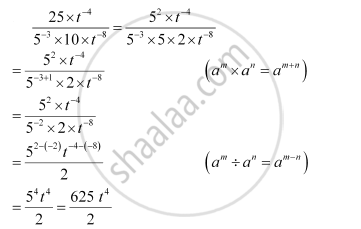# Simplify (25 Xx Tpower(-4))By(5power(-3) Xx10xxtpower(-5)) (T Not Equal 0) - Mathematics

Simplify (25 xx t^(-4))/(5^(-3) xx10xxt^(-5)) (t != 0)`

#### SolutionConcept: Powers with Negative Exponents
Is there an error in this question or solution?

#### APPEARS IN

NCERT Class 8 Maths Textbook
Chapter 12 Exponents and Powers
Exercise 12.1 | Q 7.1 | Page 198